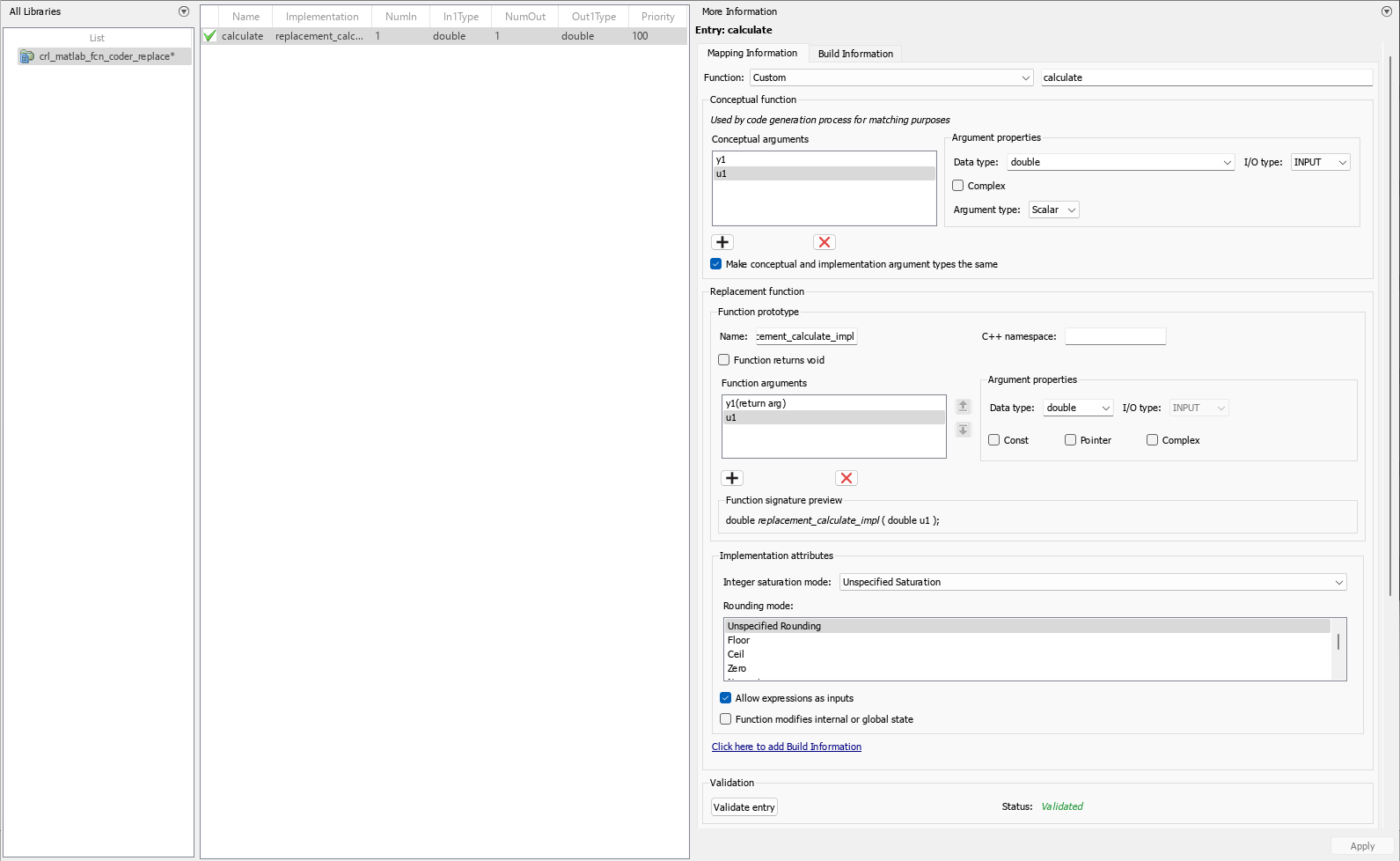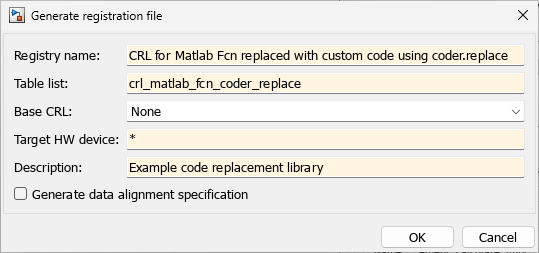## Replace MATLAB Functions with Custom Code by Using the `coder.replace` Function

You can develop a code replacement library to optimize the performance of MATLAB functions specified within a MATLAB function block containing a `coder.replace` function. To develop a code replacement library, use either the interactive or programmatic approach. For more information, see Develop a Code Replacement Library.

### Interactively Develop a Code Replacement Library

1. Open the Code Replacement Tool (crtool), from the MATLAB command line with the following command:

`>>crtool`
2. Create a table.

1. From the crtool context menu, select File > New Table.

2. In the right pane, name the table `crl_matlab_fcn_coder_replace`. Click Apply.

3. Create an entry. From the crtool context menu, select File > New entry > Function.

4. Create entry parameters. In the Function drop-down list, select `custom`. Function information appears in the crtool. For this example, specify the parameter as `calculate`.

5. Create the conceptual representation. The conceptual representation describes the signature of the function that you want to replace. In the Conceptual function subsection of the crtool, specify the return argument, y1, and the input argument, u1, with the Data Type of double and the Argument Type of Scalar.

6. Create the implementation representation. The implementation representation describes the signature of the optimization function. For this example, to specify that the implementation arguments have the same order and properties as the conceptual arguments, select the Make conceptual and implementation argument types the same check box.

Add the return argument `y1` and the arguments `u1`.

Specify a Name for the replacement function under Function prototype.

7. Specify build information. Click the Build Information tab to open the build requirements pane. Specify the files (source, header, object) that the code generator requires for code replacement. For this example, you do not need to specify build information.

8. Validate and save the table. Click the Mapping Information tab and verify the fields are filled in as shown. Click Validate entry. In the crtool context menu, select File > Save table > Save.9. Register a code replacement library. Registration creates a library composed of the tables that you specify. Select File > Generate registration file. In the Generate registration file dialog box, fill out these fields:To use your code replacement library, refresh your current MATLAB session with the command:

`>>sl_refresh_customizations`

10. Verify the code replacement library. From the MATLAB command line, open the library by using the Code Replacement Viewer and verify that the table and entry are correctly specified. For more information, see Verify Code Replacement Library. Configure your model to use the code replacement library, generate code, and verify that replacement occurs as expected. If unexpected behavior occurs, examine the hit and miss logs to troubleshoot the issues.

### Programmatically Develop a Code Replacement Library

1. Open the programmatic interface from the MATLAB menu by selecting New > Function.

2. Create a table.

1. Create a function with the name of your code replacement library table that does not have arguments and returns a table object. You can use this function to call your code replacement library table.

2. Create a table object by calling `RTW.TflTable`.

```function hTable = crl_matlab_fcn_coder_replace % Create a function to call the code replacement library table %% Create a table object hTable = RTW.TflTable;```
3. Create an entry. Because this example replaces a function, create a code replacement entry in your table by calling the entry function `RTW.TflCFunctionEntry`.

```function hTable = crl_matlab_fcn_coder_replace % Create a function to call the code replacement library table %% Create a table object hTable = RTW.TflTable; %% Create an entry hEntry = RTW.TflCFunctionEntry;```
4. Create entry parameters. Because this examples replaces a function, create entry parameters by calling the function `setTflCFunctionEntryParameters`.

```function hTable = crl_matlab_fcn_coder_replace % Create a function to call the code replacement library table %% Create a table object hTable = RTW.TflTable; %% Create an entry hEntry = RTW.TflCFunctionEntry; %% Create entry parameters setTflCFunctionEntryParameters(hEntry, ... 'Key', 'calculate', ... 'Priority', 100, ... 'ArrayLayout', 'COLUMN_MAJOR', ... 'ImplementationName', ... 'replacement_calculate_impl', ... 'ImplementationHeaderFile', ... 'replacement_calculate_impl.h', ... 'ImplementationSourceFile', ... 'replacement_calculate_impl.c');```
5. Create the conceptual representation. The conceptual representation describes the signature of the function that you want to replace. To explicitly specify argument properties, call the function `getTflArgFromString`.

```function hTable = crl_matlab_fcn_coder_replace % Create a function to call the code replacement library table %% Create a table object hTable = RTW.TflTable; %% Create an entry hEntry = RTW.TflCFunctionEntry; %% Create entry parameters setTflCFunctionEntryParameters(hEntry, ... 'Key', 'calculate', ... 'Priority', 100, ... 'ArrayLayout', 'COLUMN_MAJOR', ... 'ImplementationName', ... 'replacement_calculate_impl', ... 'ImplementationHeaderFile', ... 'replacement_calculate_impl.h', ... 'ImplementationSourceFile', ... 'replacement_calculate_impl.c'); %% Create the conceptual representation arg = getTflArgFromString(hEntry, 'y1','double'); arg.IOType = 'RTW_IO_OUTPUT'; addConceptualArg(hEntry, arg); arg = getTflArgFromString(hEntry, 'u1','double'); addConceptualArg(hEntry, arg);```
6. Create the implementation representation. The implementation representation describes the signature of the optimization function. To specify that the implementation arguments have the same order and properties as the conceptual arguments, call the function `getTflArgFromString`.

```function hTable = crl_matlab_fcn_coder_replace % Create a function to call the code replacement library table %% Create a table object hTable = RTW.TflTable; %% Create an entry hEntry = RTW.TflCFunctionEntry; %% Create entry parameters setTflCFunctionEntryParameters(hEntry, ... 'Key', 'calculate', ... 'Priority', 100, ... 'ArrayLayout', 'COLUMN_MAJOR', ... 'ImplementationName', ... 'replacement_calculate_impl', ... 'ImplementationHeaderFile', ... 'replacement_calculate_impl.h', ... 'ImplementationSourceFile', ... 'replacement_calculate_impl.c'); %% Create the conceptual representation arg = getTflArgFromString(hEntry, 'y1','double'); arg.IOType = 'RTW_IO_OUTPUT'; addConceptualArg(hEntry, arg); arg = getTflArgFromString(hEntry, 'u1','double'); addConceptualArg(hEntry, arg); %% Create the Conceptual Representation arg = getTflArgFromString(hEntry, 'y1','double'); arg.IOType = 'RTW_IO_OUTPUT'; hEntry.Implementation.setReturn(arg); arg = getTflArgFromString(hEntry, 'u1','double'); hEntry.Implementation.addArgument(arg); %% Add the entry to the table hTable.addEntry(hEntry);```
7. Specify build information. In the entry parameters, specify files (header, source, object) that the code generator needs for code replacement. For this example, build information is not required.

8. Validate and save the customization file. From the MATLAB menu, save this customization file by selecting File > Save. From the command line, validate the code replacement library table by calling it:

`>> hTable = crl_matlab_fcn_coder_replace`
9. Register the code replacement library. Registration creates a code replacement library by defining the library name, code replacement tables, and other information. Create a registration file by using these specifications:

```function rtwTargetInfo(cm) cm.registerTargetInfo(@loc_register_crl); end function this = loc_register_crl this(1) = RTW.TflRegistry; this(1).Name = 'CRL for matlab function with custom code using coder.replace’; this(1).TableList = {'crl_matlab_fcn_coder_replace.m'}; % table created in this example this(1).TargetHWDeviceType = {'*'}; this(1).Description = 'Example code replacement library'; end ```

To use your code replacement library, refresh your current MATLAB session with the command:

`>>sl_refresh_customizations`

10. Verify the code replacement library. From the MATLAB command line, open the library by using the Code Replacement Viewer and verify that the table and entry are correctly specified. For more information, see Verify Code Replacement Library. Configure your model to use the code replacement library, generate code, and verify that replacement occurs as expected. If unexpected behavior occurs, examine the hit and miss logs to troubleshoot the issues.